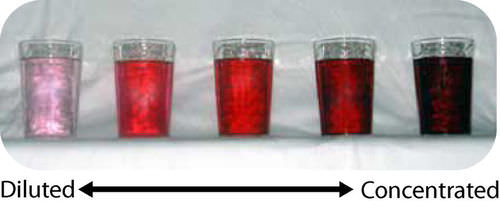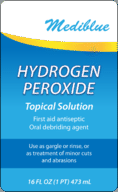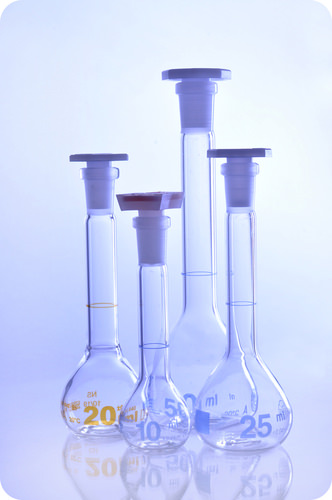# 8.1: Concentrations of Solutions

$$\newcommand{\vecs}{\overset { \rightharpoonup} {\mathbf{#1}} }$$ $$\newcommand{\vecd}{\overset{-\!-\!\rightharpoonup}{\vphantom{a}\smash {#1}}}$$$$\newcommand{\id}{\mathrm{id}}$$ $$\newcommand{\Span}{\mathrm{span}}$$ $$\newcommand{\kernel}{\mathrm{null}\,}$$ $$\newcommand{\range}{\mathrm{range}\,}$$ $$\newcommand{\RealPart}{\mathrm{Re}}$$ $$\newcommand{\ImaginaryPart}{\mathrm{Im}}$$ $$\newcommand{\Argument}{\mathrm{Arg}}$$ $$\newcommand{\norm}{\| #1 \|}$$ $$\newcommand{\inner}{\langle #1, #2 \rangle}$$ $$\newcommand{\Span}{\mathrm{span}}$$ $$\newcommand{\id}{\mathrm{id}}$$ $$\newcommand{\Span}{\mathrm{span}}$$ $$\newcommand{\kernel}{\mathrm{null}\,}$$ $$\newcommand{\range}{\mathrm{range}\,}$$ $$\newcommand{\RealPart}{\mathrm{Re}}$$ $$\newcommand{\ImaginaryPart}{\mathrm{Im}}$$ $$\newcommand{\Argument}{\mathrm{Arg}}$$ $$\newcommand{\norm}{\| #1 \|}$$ $$\newcommand{\inner}{\langle #1, #2 \rangle}$$ $$\newcommand{\Span}{\mathrm{span}}$$

Learning Outcomes

• Define concentration.
• Use the terms concentrated and dilute to describe the relative concentration of a solution.
• Calculate the molarity of a solution.
• Calculate percentage concentration (m/m, v/v, m/v).
• Describe a solution whose concentration is in $$\text{ppm}$$ or $$\text{ppb}$$.
• Use concentration units in calculations.
• Determine equivalents for an ion.
• Complete calculations relating equivalents to moles, volumes, or mass.
• Complete dilution calculations.

There are several ways to express the amount of solute present in a solution. The concentration of a solution is a measure of the amount of solute that has been dissolved in a given amount of solvent or solution. A concentrated solution is one that has a relatively large amount of dissolved solute. A dilute solution is one that has a relatively small amount of dissolved solute. However, these terms are relative, and we need to be able to express concentration in a more exact, quantitative manner. Still, concentrated and dilute are useful as terms to compare one solution to another (see figure below). Also, be aware that the terms "concentrate" and "dilute" can be used as verbs. If you were to heat a solution, causing the solvent to evaporate, you would be concentrating it, because the ratio of solute to solvent would be increasing. If you were to add more water to an aqueous solution, you would be diluting it because the ratio of solute to solvent would be decreasing.Figure $$\PageIndex{1}$$: Solutions of a red dye in water from the most dilute (on the left) to the most concentrated (on the right).

## Percent Concentration

One way to describe the concentration of a solution is by the percent of the solution that is composed of the solute. This percentage can be determined in one of three ways: (1) the mass of the solute divided by the mass of solution, (2) the volume of the solute divided by the volume of the solution, or (3) the mass of the solute divided by the volume of the solution. Because these methods generally result in slightly different vales, it is important to always indicate how a given percentage was calculated.

### Mass Percent

When the solute in a solution is a solid, a convenient way to express the concentration is a mass percent (mass/mass), which is the grams of solute per $$100 \: \text{g}$$ of solution.

$\text{Percent by mass} = \frac{\text{mass of solute}}{\text{mass of solution}} \times 100\%$

Suppose that a solution was prepared by dissolving $$25.0 \: \text{g}$$ of sugar into $$100 \: \text{g}$$ of water. The percent by mass would be calculated as follows:

$\text{Percent by mass} = \frac{25 \: \text{g sugar}}{125 \: \text{g solution}} \times 100\% = 20\% \: \text{sugar}$

Sometimes, you may want to make a particular amount of solution with a certain percent by mass and will need to calculate what mass of the solute is needed. For example, let's say you need to make $$3.00 \times 10^3 \: \text{g}$$ of a sodium chloride solution that is $$5.00\%$$ by mass. You can rearrange and solve for the mass of solute.

\begin{align} \% \: \text{by mass} &= \frac{\text{mass of solute}}{\text{mass of solution}} \times 100\% \\ 5.00\% &= \frac{\text{mass of solute}}{3.00 \times 10^3 \: \text{g solution}} \times 100\% \\ \text{mass of solute} &= 150. \: \text{g} \end{align}

You would need to weigh out $$150 \: \text{g}$$ of $$\ce{NaCl}$$ and add it to $$2850 \: \text{g}$$ of water. Notice that it was necessary to subtract the mass of the $$\ce{NaCl}$$ $$\left( 150 \: \text{g} \right)$$ from the mass of solution $$\left( 3.00 \times 10^3 \: \text{g} \right)$$ to calculate the mass of the water that would need to be added.

### Volume Percent

The percentage of solute in a solution can more easily be determined by volume when the solute and solvent are both liquids. The volume of the solute divided by the volume of the solution expressed as a percent, yields the percent by volume (volume/volume) of the solution. If a solution is made by taking $$40. \: \text{mL}$$ of ethanol and adding enough water to make $$240. \: \text{mL}$$ of solution, the percent by volume is:

\begin{align} \text{Percent by volume} &= \frac{\text{volume of solute}}{\text{volume of solution}} \times 100\% \\ &= \frac{40 \: \text{mL ethanol}}{240 \: \text{mL solution}} \times 100\% \\ &= 16.7\% \: \text{ethanol} \end{align}

Frequently, ingredient labels on food products and medicines have amounts listed as percentages (see figure below).Figure $$\PageIndex{2}$$: Hydrogen peroxide is commonly sold as a $$3\%$$ by volume solution for use as a disinfectant.

It should be noted that, unlike in the case of mass, you cannot simply add together the volumes of solute and solvent to get the final solution volume. When adding a solute and solvent together, mass is conserved, but volume is not. In the example above, a solution was made by starting with $$40 \: \text{mL}$$ of ethanol and adding enough water to make $$240 \: \text{mL}$$ of solution. Simply mixing $$40 \: \text{mL}$$ of ethanol and $$200 \: \text{mL}$$ of water would not give you the same result, as the final volume would probably not be exactly $$240 \: \text{mL}$$.

The mass-volume percent is also used in some cases and is calculated in a similar way to the previous two percentages. The mass/volume percent is calculated by dividing the mass of the solute by the volume of the solution and expressing the result as a percent.

For example, if a solution is prepared from $$10 \: \ce{NaCl}$$ in enough water to make a $$150 \: \text{mL}$$ solution, the mass-volume concentration is

\begin{align} \text{Mass-volume concentration} & \frac{\text{mass solute}}{\text{volume solution}} \times 100\% \\ &= \frac{10 \: \text{g} \: \ce{NaCl}}{150 \: \text{mL solution}} \times 100\% \\ &= 6.7\% \end{align}

### Parts per Million and Parts per Billion

Two other concentration units are parts per million and parts per billion. These units are used for very small concentrations of solute such as the amount of lead in drinking water. Understanding these two units is much easier if you consider a percentage as parts per hundred. Remember that $$85\%$$ is the equivalent of 85 out of a hundred. A solution that is $$15 \: \text{ppm}$$ is 15 parts solute per 1 million parts solution. A $$22 \: \text{ppb}$$ solution is 22 parts solute per billion parts solution. While there are several ways of expressing two units of $$\text{ppm}$$ and $$\text{ppb}$$, we will treat them as $$\text{mg}$$ or $$\mu \text{g}$$ of solutes per $$\text{L}$$ solution, respectively.

For example, $$32 \: \text{ppm}$$ could be written as $$\frac{32 \: \text{mg solute}}{1 \: \text{L solution}}$$ while $$59 \: \text{ppb}$$ can be written as $$\frac{59 \: \mu \text{g solute}}{1 \: \text{L solution}}$$.

## Molarity

Chemists primarily need the concentration of solutions to be expressed in a way that accounts for the number of particles present that could react according to a particular chemical equation. Since percentage measurements are based on either mass or volume, they are generally not useful for chemical reactions. A concentration unit based on moles is preferable. The molarity $$\left( \text{M} \right)$$ of a solution is the number of moles of solute dissolved in one liter of solution. To calculate the molarity of a solution, you divide the moles of solute by the volume of the solution expressed in liters.

$\text{Molarity} \: \left( \text{M} \right) = \frac{\text{moles of solute}}{\text{liters of solution}} = \frac{\text{mol}}{\text{L}}$

Note that the volume is in liters of solution and not liters of solvent. When a molarity is reported, the unit is the symbol $$\text{M}$$, which is read as "molar". For example, a solution labeled as $$1.5 \: \text{M} \: \ce{NH_3}$$ is a "1.5 molar solution of ammonia".

Example $$\PageIndex{1}$$

A solution is prepared by dissolving $$42.23 \: \text{g}$$ of $$\ce{NH_4Cl}$$ into enough water to make $$500.0 \: \text{mL}$$ of solution. Calculate its molarity.

Solution

Step 1: List the known quantities and plan the problem.

Known

• Mass of $$\ce{NH_4Cl} = 42.23 \: \text{g}$$
• Molar mass of $$\ce{NH_4Cl} = 53.50 \: \text{g/mol}$$
• Volume of solution $$= 500.0 \: \text{mL} = 0.5000 \: \text{L}$$

Unknown

• Molarity $$= ? \: \text{M}$$

The mass of the ammonium chloride is first converted to moles. Then, the molarity is calculated by dividing by liters. Note that the given volume has been converted to liters.

Step 2: Solve.

$42.23 \: \text{g} \: \ce{NH_4Cl} \times \frac{1 \: \text{mol} \: \ce{NH_4Cl}}{53.50 \: \text{g} \: \ce{NH_4Cl}} = 0.7893 \: \text{mol} \: \ce{NH_4Cl}$

$\frac{0.7893 \: \text{mol} \: \ce{NH_4Cl}}{0.5000 \: \text{L}} = 1.579 \: \text{M}$

The molarity is $$1.579 \: \text{M}$$, meaning that a liter of the solution would contain 1.579 moles of $$\ce{NH_4Cl}$$. Having four significant figures is appropriate.Figure $$\PageIndex{3}$$: Volumetric flasks come in many sizes, each designed to prepare a different volume of solution.

## Dilutions

When additional water is added to an aqueous solution, the concentration of that solution decreases. This is because the number of moles of the solute does not change, but the total volume of the solution increases. We can set up an equality between the moles of the solute before the dilution (1) and the moles of the solute after the dilution (2).

$\text{mol}_1 = \text{mol}_2$

Since the moles of solute in a solution is equal to the molarity multiplied by the volume in liters, we can set those equal.

$M_1 \times L_1 = M_2 \times L_2$

Finally, because the two sides of the equation are set equal to one another, the volume can be in any units we choose, as long as that unit is the same on both sides. Our equation for calculating the molarity of a diluted solution becomes:

$M_1 \times V_1 = M_2 \times V_2$

Additionally, the concentration can be in any other unit as long as $$M_1$$ and $$M_2$$ are in the same unit.

Suppose that you have $$100. \: \text{mL}$$ of a $$2.0 \: \text{M}$$ solution of $$\ce{HCl}$$. You dilute the solution by adding enough water to make the solution volume $$500. \: \text{mL}$$. The new molarity can easily be calculated by using the above equation and solving for $$M_2$$.

$M_2 = \frac{M_1 \times V_1}{V_2} = \frac{2.0 \: \text{M} \times 100. \: \text{mL}}{500. \: \text{mL}} = 0.40 \: \text{M} \: \ce{HCl}$

The solution has been diluted by a factor of five, since the new volume is five times as great as the original volume. Consequently, the molarity is one-fifth of its original value. Another common dilution problem involves deciding how much a highly concentrated solution is required to make a desired quantity of solution with a lower concentration. The highly concentrated solution is typically referred to as the stock solution.

Example $$\PageIndex{2}$$

Nitric acid $$\left( \ce{HNO_3} \right)$$ is a powerful and corrosive acid. When ordered from a chemical supply company, its molarity is $$16 \: \text{M}$$. How much of the stock solution of nitric acid needs to be used to make $$8.00 \: \text{L}$$ of a $$0.50 \: \text{M}$$ solution?

Solution

Step 1: List the known quantities and plan the problem.

Known

• Stock $$\ce{HNO_3} \: \left( M_1 \right) = 16 \: \text{M}$$
• $$V_2 = 8.00 \: \text{L}$$
• $$M_2 = 0.50 \: \text{M}$$

Unknown

• Volume of stock $$\ce{HNO_3} \: \left( V_1 \right) = ? \: \text{L}$$

The unknown in the equation is $$V_1$$, the necessary volume of the concentrated stock solution.

Step 2: Solve.

$V_1 = \frac{M_2 \times V_2}{V_1} = \frac{0.50 \: \text{M} \times 8.00 \: \text{L}}{16 \: \text{M}} = 0.25 \: \text{L} = 250 \: \text{mL}$

$$250 \: \text{mL}$$ of the stock $$\ce{HNO_3}$$ solution needs to be diluted with water to a final volume of $$8.00 \: \text{L}$$. The dilution from $$16 \: \text{M}$$ to $$0.5 \: \text{M}$$ is a factor of 32.

## Equivalents

Concentration is important in healthcare because it is used in so many ways. It's also critical to use units with any values to ensure the correct dosage of medications or report levels of substances in blood, to name just two.

Another way of looking at concentration such as in IV solutions and blood is in terms of equivalents. One equivalent is equal to one mole of charge in an ion. The value of the equivalents is always positive regardless of the charge. For example, $$\ce{Na^+}$$ and $$\ce{Cl^-}$$ both have 1 equivalent per mole.

$\begin{array}{ll} \textbf{Ion} & \textbf{Equivalents} \\ \ce{Na^+} & 1 \\ \ce{Mg^{2+}} & 2 \\ \ce{Al^{3+}} & 3 \\ \ce{Cl^-} & 1 \\ \ce{NO_3^-} & 1 \\ \ce{SO_4^{2-}} & 2 \end{array}$

Equivalents are used because the concentration of the charges is important than the identity of the solutes. For example, a standard IV solution does not contain the same solutes as blood but the concentration of charges is the same.

Sometimes, the concentration is lower in which case milliequivalents $$\left( \text{mEq} \right)$$ is a more appropriate unit. Just like metric prefixes used with base units, milli is used to modify equivalents so $$1 \: \text{Eq} = 1000 \: \text{mEq}$$.

Example $$\PageIndex{3}$$

How many equivalents of $$\ce{Ca^{2+}}$$ are present in a solution that contains 3.5 moles of $$\ce{Ca^{2+}}$$?

Solution

Use the relationship between moles and equivalents of $$\ce{Ca^{2+}}$$ to find the answer.

$3.5 \: \text{mol} \cdot \frac{2 \: \text{Eq}}{1 \: \text{mol} \: \ce{Ca^{2+}}} = 7.0 \: \text{Eq} \: \ce{Ca^{2+}}$

Example $$\PageIndex{4}$$

A patient received $$1.50 \: \text{L}$$ of saline solution which has a concentration of $$154 \: \text{mEq/L} \: \ce{Na^+}$$. What mass of sodium did the patient receive?

Solution

Use dimensional analysis to set up the problem based on the values given in the problem, the relationship for $$\ce{Na^+}$$ and equivalents and the molar mass of sodium. Note that if this problem had a different ion with a different charge, that would need to be accounted for in the calculation.

$1.50 \: \text{L} \cdot \frac{154 \: \text{mEq}}{1 \: \text{L}} \cdot \frac{1 \: \text{Eq}}{1000 \: \text{mEq}} \cdot \frac{1 \: \text{mol} \: \ce{Na^+}}{1 \: \text{Eq}} \cdot \frac{22.99 \: \text{g}}{1 \: \text{mol} \: \ce{Na^+}} = 5.31 \: \text{g} \: \ce{Na^+}$